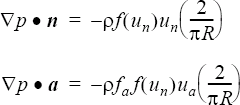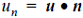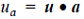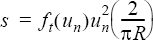# LINE_SOURCE

Specifies sources on a line.

AcuSolve Command

## Syntax

LINE_SOURCE("name"){parameters...}

User-given name.

## Parameters

lines (array) [no default]
A three-column array defining the line connectivity.
friction_type (enumerated) [=none]
Type of the friction factor.
none
No friction.
constant or const
Constant for the entire set. Requires friction.
piecewise_linear or linear
Piecewise linear curve fit. Requires friction_curve_fit_values and friction_curve_fit_variable.
cubic_spline or spline
Cubic spline curve fit. Requires friction_curve_fit_values and friction_curve_fit_variable.
friction (real) >=0 [=1]
Constant value of the friction factor. Used with constant friction type.
friction_curve_fit_values (array) [={0,1}]
A two-column array of independent-variable/friction-factor data values. Used with piecewise_linear and cubic_spline friction types.
friction_curve_fit_variable (enumerated) [=normal_velocity]
Independent variable of the friction factor curve fit. Used with piecewise_linear and cubic_spline friction types.
normal_velocity
Velocity component normal to the line.
friction_multiplier_function (string) [=none]
User-given name of the multiplier function for scaling the friction factor. If none, no scaling is performed.
axial_friction_factor (real) >=0 [=10]
Fraction of the friction factor used for the axial friction factor.
volume_heat_source_type (enumerated) [=none]
Type of the heat source per unit volume.
none
No volume heat source.
constant or const
Constant for the entire set. Requires volume_heat_source.
volume_heat_source (real) >=0 [=1]
Constant value of the heat source per unit volume. Used with constant volume heat source type.
volume_heat_source_multiplier_function (string) [=none]
User-given name of the multiplier function for scaling the volume heat source. If none, no scaling is performed.
turbulence_source_type (enumerated) [=none]
Type of the turbulence source.
none
No turbulence source.
constant or const
Constant for the entire set. Requires turbulence_source.
piecewise_linear or linear
Piecewise linear curve fit. Requires turbulence_source_curve_fit_values and turbulence_source_curve_fit_variable.
cubic_spline or spline
Cubic spline curve fit. Requires turbulence_source_curve_fit_values and turbulence_source_curve_fit_variable.
turbulence_source (real) >=0 [=0]
Constant value of the turbulence source. Used with constant turbulence source type.
turbulence_source_curve_fit_values (array) [={0,1}]
A two-column array of independent-variable/turbulence-source data values. Used with piecewise_linear and cubic_spline turbulence source types.
turbulence_source_curve_fit_variable (enumerated) [=normal_velocity]
Independent variable of the turbulence source curve fit. Used with piecewise_linear and cubic_spline turbulence source types.
normal_velocity
Velocity component normal to the line.
turbulence_source_multiplier_function (string) [=none]
User-given name of the multiplier function for scaling the turbulence source. If none, no scaling is performed.
mesh_motion (string) [=none]
User-given name of the MESH_MOTION command for specifying mesh displacement boundary conditions on all the nodes in this line. If none, no boundary conditions are imposed.

## Description

This command specifies momentum, heat, and turbulence sources on a line.

For example,
LINE_SOURCE( "example line source" ) {
lines                                   = { 1001, 1, 2 ;
1002, 3, 4 ;
1003, 5, 6 ; }
friction_type                           = constant
friction                                = 1
axial_friction_factor                   = 10
friction_multiplier_function            = none
volume_heat_source_type                 = constant
volume_heat_source                      = 2
volume_heat_source_multiplier_function  = none
turbulence_source_type                  = constant
turbulence_source                       = 0.01
turbulence_source_multiplier_function   = none
mesh_motion                             = none
}
creates sources along a line for the momentum, temperature, and turbulence equations. If any equation is not defined, then the corresponding source is ignored. The effective radius of the line is 1. The lines parameter is mandatory. It is a three-column array corresponding to a unique (within this set) line number, and the two nodes (in the global coordinates list) of a line segment. The lines may be read from a file. For the above example the lines may be placed in a file, such as example.lin:
1001      1         2
1002       3         4
1003      5         6
LINE_SOURCE( "example line source" ) {
...
}
The force on the line is defined in terms of the pressure drop in the normal and axial directions:(1)where p is the fluid pressure; a is the direction parallel to the line; n is the direction of the fluid velocity projected to the plane normal to the line (that is, the "normal" direction); f(un) is the friction factor, given by friction_type and related parameters (see below); faf(un) is the axial friction factor; fa is given by axial_friction_factor; R is the effective radius, given by radius;is the normal velocity;is the axial velocity; and u is the fluid velocity vector. The pressure drop is integrated over the effective volume yielding a force on the fluid, which is applied only at the nodes on the line.

If friction_type=constant, the friction factor is given by friction.

A piecewise_linear or cubic_spline friction type may be used to define the friction factor as a function of a single independent variable. The only independent variable currently supported is the normal velocity. For example,
LINE_SOURCE( "curve fit friction factor" ) {
friction_type               = piecewise_linear
friction_curve_fit_values   = { 0, 10.685 ;
5, 3.291 ;
10, 1.996 ;
15, 1.495 ;
20, 1.220 ;
30, 0.920 ;
40, 0.756 ;
50, 0.650 ; }
friction_curve_fit_variable = normal_velocity
...
}

defines the friction factor as a function of the normal velocity (that is, velocity in the direction of the heat exchanger). The friction_curve_fit_values parameter is a two-column array corresponding to the independent variable and the friction factor. The independent variable values must be in ascending order. The limit point values of the curve fit are used when friction_curve_fit_variable falls outside of the curve fit limits.

The friction_curve_fit_values data may be read from a file. For the above example, the curve fit values may be placed in a file, such as friction.fit:
0    10.685
5     3.291
10    1.996
15    1.495
20    1.220
30    0.920
40    0.756
50    0.650
LINE_SOURCE( "curve fit friction factor" ) {
friction_type                = piecewise_linear
friction_curve_fit_variable  = normal_velocity
...
}

A volumetric heat source for the temperature equation is given by volume_heat_source. This has the same units and effect as the VOLUME_HEAT_SOURCE command. The only difference is that here the heat source is integrated over the effective volume and applied only on the nodes of the line.

For the Spalart-Allmaras and Detached Eddy Simulation turbulence models, a turbulence line source is given by:(2)where ft(un) is the turbulence source, given by turbulence_source_type and related parameters; and s is an effective volumetric source term in the turbulence model equation. The latter is integrated over the effective volume and applied only on the nodes of the line.

If turbulence_source_type=constant, the turbulence source is given by turbulence_source. A piecewise_linear or cubic_spline turbulence source type may be used to define the turbulence source as a function of the normal velocity.

The friction_multiplier_function, volume_heat_source_multiplier_function and turbulence_source_multiplier_function parameters may be used to scale the friction factor, volume heat source, and turbulence source, respectively. The value of each of these parameters refers to the user given name of a MULTIPLIER_FUNCTION command in the input file.

The mesh_motion parameter is provided as a shortcut way to impose nodal boundary conditions on all three components of the mesh displacement. The parameter refers to a MESH_MOTION command where the motion is defined. Specifying mesh_motion is equivalent to providing three NODAL_BOUNDARY_CONDITION commands on the components of the mesh displacement, with each command referring to all the nodes in the line connectivity, setting precedence=0, and including the same mesh_motion parameter. If mesh=eulerian in the EQUATION command or mesh_motion=none then this parameter has no effect. For example, a line that rotates with a fan may be defined by:
LINE_SOURCE( "rotating fan" ) {
mesh_motion        = "rotating fan"
...
}
MESH_MOTION( "rotating fan" ) {
type               = rotation
rotation_center    = { 0, 0, 0 }
angular_velocity   = { 0, 3, 0 }
}